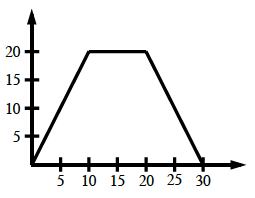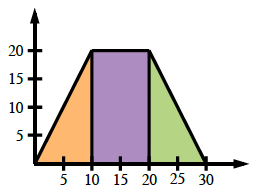### Home > PC > Chapter 9 > Lesson 9.3.2 > Problem9-111

9-111.
1. Graph the velocity (in ft/s) according to the following function: Homework Help ✎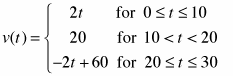1. What would the area under the curve represent?

2. Calculate the area under the curve.

3. How does this relate to a position graph?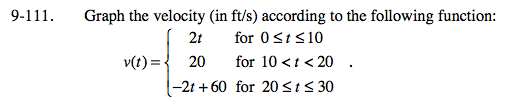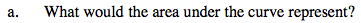The area under the curve represents distance traveled.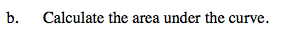Be careful of scale and units!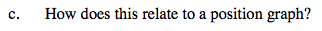If p = 0 when t = 0, then p = 400 when t = 30.